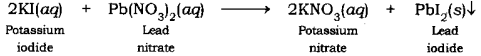# (i) What is observed when a solution of potassium iodide is added to a solution of lead nitrate taken in a test tube?

(i) What is observed when a solution of potassium iodide is added to a solution of lead nitrate taken in a test tube?
(ii) What type of reaction is this?
(iii) Write a balanced chemical equation to represent the above reaction.

(i) When a solution of potassium iodide is added to a solution of lead nitrate taken in a test tube, the precipitation of a yellowish solid is observed. This yellowish solid is lead iodide. Potassium nitrate is formed along with lead iodide.

(ii) This is a double displacement reaction.

(in) The balanced chemical equation for the above reaction is: